基于BMA方法的地面气温概率预报研究Probabilistic Forecast of Surface Air Temperature Using Bayesian Model Averaging

• 全文下载: PDF(2385KB)    PP.269-278   DOI: 10.12677/CCRL.2019.83030
• 下载量: 110  浏览量: 156

In this study, based on European Centre for Mediu-Range Weather Forecasts (ECMWF) and Global Forecast System (GFS), the probabilistic forecasts of surface air temperature during the period from 1 June to 31 November 2018 were conducted using Bayesian Model Averaging (BMA). The results showed that BMA method can not only provide deterministic result prediction, but also provide full probability density function to describe the uncertainty of prediction quantitatively. By using Analyzed the Correlation Coefficient (ACC), Root-mean-square error (RMSE) and the prediction accuracy of the prediction errors at 1˚C and 2˚C, the prediction results of BMA are better than models. The continuous ranked probability score (CRPS) is used to evaluate the probability prediction of BMA, and it is found that the probability prediction skills of BMA are also improved. In addition, for the probability forecast provided by BMA, the temperature forecast under 80% probability can be used as the limit of the maximum temperature forecast.

1. 引言

2. 资料和方法

2.1. 资料Table 1. Basic characteristics of the two climate models

2.2. 方法

2.2.1. 贝叶斯模式平均

BMA是一种集合预报的前处理方法，可以产生有预测效果的概率预报，也可以产生确定性预报。它以实测样本隶属于某一模式的后验概率为权重，将各模式的条件概率密度函数进行加权平均，从而得到概率密度函数，其基本原理如下：

$p\left(y|\left({f}_{1},{f}_{2},\cdots ,{f}_{K},{y}^{\text{T}}\right)\right)=\underset{k=1}{\overset{K}{\sum }}{\omega }_{k}{h}_{k}\left(y|\left({f}_{k},{y}^{\text{T}}\right)\right)$

${h}_{k}\left(y|\left({f}_{k},{y}^{\text{T}}\right)\right)$ 是与单个模式回报结果 ${f}_{k}$ 相联系的条件概率密度函数。

BMA模型的预报方差包括两项，BMA模型的预报方差包括两项，第一项是模式间的方差，反映了预报集合的离散度，第二项表示集合内的方差。

$\mathrm{var}\left[y|\left({f}_{1st},{f}_{2st},\cdots ,{f}_{Kst},{y}^{\text{T}}\right)\right]=\underset{k=1}{\overset{K}{\sum }}{w}_{k}{\left(\left({a}_{k}+{b}_{k}{f}_{kst}\right)-\underset{k=1}{\overset{K}{\sum }}{w}_{l}\left({a}_{k}+{b}_{k}{f}_{lst}\right)\right)}^{2}+{\sigma }^{2}$

2.2.2. Cressman插值

Cressman插值算法由Cressman等  在1959年提出，采用逐步订正的方法进行最优化插值，用实际资料与预备场或者初值场之差去改变和订正预备场或者初值场，得到一个新场，再求出新场与实际值之差，去订正上一次的场，直到订正后的场逼近观测记录为止。

Cressman插值算法的基本公式模型为：

${\alpha }^{\prime }={\alpha }_{0}+\Delta {\alpha }_{ij}$

$\Delta {\alpha }_{ij}=\frac{{\sum }_{k=1}^{K}\left({W}_{ijk}^{2}\Delta {\alpha }_{k}\right)}{{\sum }_{k=1}^{K}\text{ }{W}_{ijk}}$

Cressman插值最重要的是权重函数 ${W}_{ijk}$ 的确定，其一般形式为：

${W}_{ijk}=\left\{\begin{array}{l}\frac{{R}^{2}-{d}_{ijk}^{2}}{{R}^{2}+{d}_{ijk}^{2}},\text{\hspace{0.17em}}\text{\hspace{0.17em}}\text{\hspace{0.17em}}\text{\hspace{0.17em}}\text{ }\text{ }{d}_{ijk}

Cressman插值算法的基本思路如下：

S1：需要确定一个预备场，并设定一个逼近值范围，用于比较计算后的新值与实际资料的差值；

S2：计算权重 ${W}_{ijk}$

S3：将权重 ${W}_{ijk}$ 放入插值计算中，得到一个新场；

S4：将这个新场与实际资料相比，计算他们的差是否在预定的逼近值范围内，如果不在逼近值范围内，就用这个新场与实际值的差，去订正上一次的场；

S5：对订正后的场和实际值进行比较，如果还是超过逼近值的范围，则继续订正，直到新场与实际值的差在预定的逼近值范围内。

2.3. 检验评估方法

1) 均方根误差(Root-mean-square error, RMSE)，其定义为：

$\text{RMSE}=\sqrt{\frac{1}{n}\underset{i=1}{\overset{n}{\sum }}{\left({y}_{i}-{o}_{i}\right)}^{2}}$

2) 距平相关系数(Anomaly Correlation Coefficient, ACC)，其定义为：

$\text{ACC}=\frac{\frac{1}{n}\underset{i=1}{\overset{n}{\sum }}\left({y}_{i}-\stackrel{¯}{y}\right)\left({o}_{i}-\stackrel{¯}{o}\right)}{\sqrt{\frac{1}{n}{\left({y}_{i}-\stackrel{¯}{y}\right)}^{2}\frac{1}{n}{\left({o}_{i}-\stackrel{¯}{o}\right)}^{2}}}$

3) 连续分级概率评分(Continuous Ranked Probability Score, CRPS)，其定义为：

$\text{CRPS}=\frac{1}{n}\underset{i=1}{\overset{n}{\sum }}\int {\left[f\left({y}_{i}\right)-H\left({y}_{i}-{o}_{i}\right)\right]}^{2}\text{d}x$

3. 检验评估

CRPS可以看出在所有预报时效内，BMA的预报技巧都高于其他两个模式，最高技巧提高幅度可达0.4，并且随着预报时效的增长，预报的技巧提高幅度逐渐减小。另外，对于所有时效，CRPS值还是比较大。说明各个模式以及BMA方法对盐城区域范围内2米气温预报仍存在一定的困难。也说明对盐城区域的气温的预报研究存在一定的实际意义。

4. BMA概率预报结果

4.1. 单点预报结果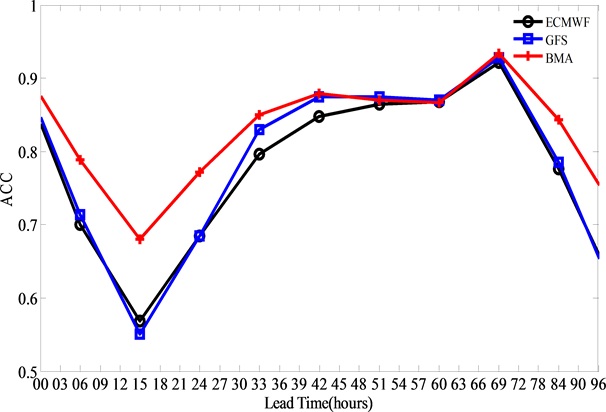Figure 1. The variation of ACC with 96 h leading time at 08:00 for T2m by different model (ECMWF, GFS) and BMA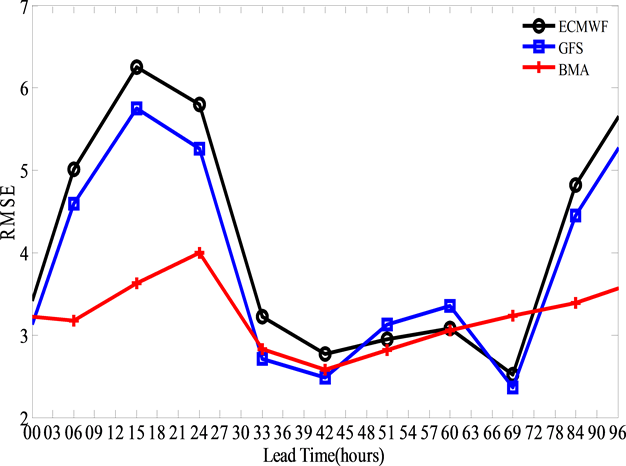Figure 2. The variation of RMSE with 96 h leading time at 08:00 for T2m by different model (ECMWF, GFS) and BMA (unit: ˚C)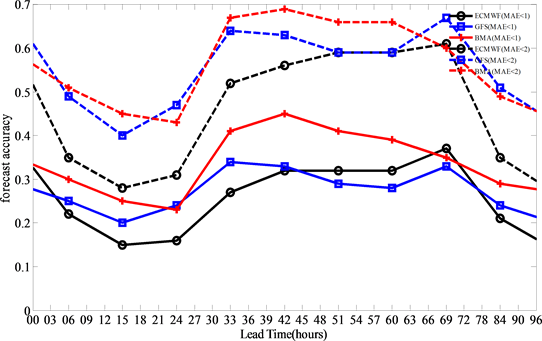Figure 3. The variation of forecast accuracy of 1˚C and 2˚C with 96 h leading time at 08:00 for T2m by different model (ECMWF, GFS) and BMA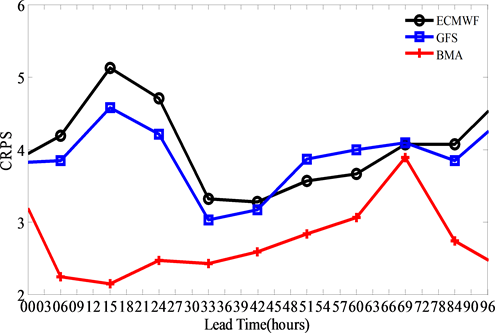Figure 4. The variation if CRPS with 96 h leading time at 08:00 for T2m by different model (ECMWF, GFS) and BMA

4.2. 区域预报结果特征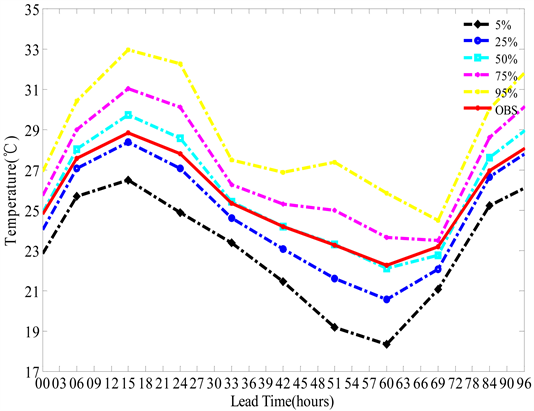Figure 5. Surface air temperature of different probability (5%, 25%, 50%, 75%, 95%) in 00 - 96 h at the grid point (32˚N, 119.45˚E), the dotted curves are the BMA predictive, dashed curve is observation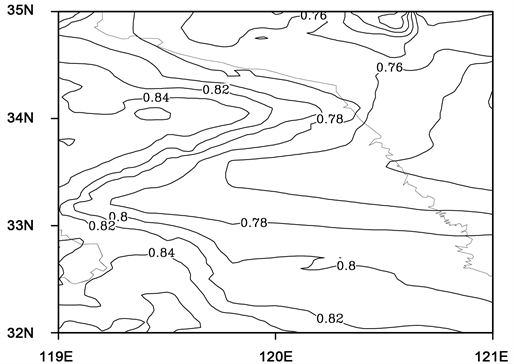Figure 6. The spatial probability distribution of the observed maximum surface air temperature with a lead time of 000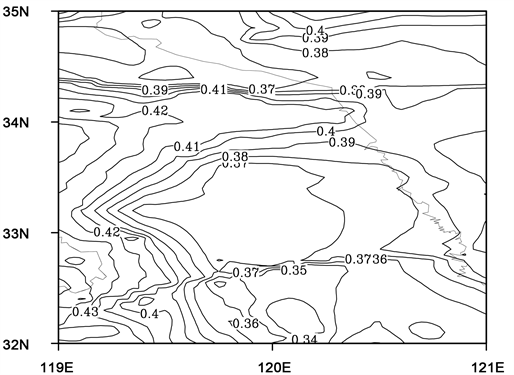Figure 7. The spatial probability distribution of the observed surface air temperature with a lead time of 000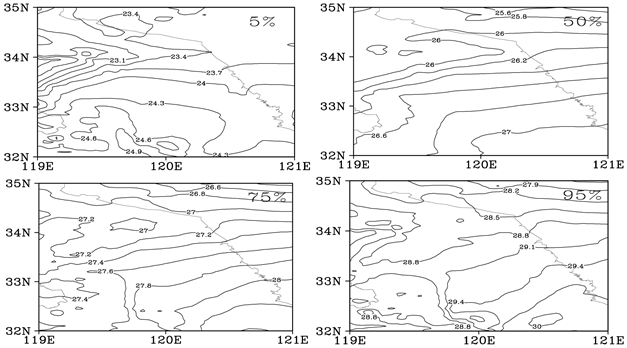Figure 8. The spatial distribution of surface air temperature in probability of 5% - 95% in leading time 000 at 08:00

5. 总结

1) BMA方法对于0~96 h预报时效不仅可以提供确定性结果也可以做出概率预报，对于确定性结果，ACC、RMSE、误差在1℃和2℃的预报准确率而言，预报技巧都有提高。对于概率预报，CRPS预报技巧都有提高。

2) BMA提供的确定性预报结果可在实际精细化预报中发挥作用。

3) BMA概率预报，提供的80%概率下的预报值可作为最高气温的预警。这对于夏季高温预警有很好的警示作用。

  Lorenz, E.N. (1963) Deterministic Non-Periodic Flow. Journal of the Atmospheric Sciences, 20, 130-141. https://doi.org/10.1175/1520-0469(1963)020<0130:DNF>2.0.CO;2  Lorenz, E.N. (1965) A Study of the Predictability of a 28-Variable Atmosphere Model. Tellus, 17, 321-333. https://doi.org/10.3402/tellusa.v17i3.9076  杜钧, 陈静. 单一值预报向概率预报转变的基础: 谈谈集合预报及其带来的变革[J]. 气象, 2010, 36(11): 1-11.  矫梅燕. 天气业务的现代化发展[J]. 气象, 2010, 36(7): 1-4.  Epstein, E.S. (1969) Stochastic Dynamic Prediction. Tellu, 21, 739-759. https://doi.org/10.3402/tellusa.v21i6.10143  杜钧, 邓国. 单一值预报向概率预报转变的价值: 谈谈概率预报的检验和应用[J]. 气象, 2010, 36(12): 10-18.  Raftery, A.E., Gneiting, T., Balabdaoui, F., et al. (2005) Using Bayesian Model Averaging to Calibrate Forecast Ensembles. Monthly Weather Review, 133. https://doi.org/10.1175/MWR2906.1  智协飞, 彭婷, 王玉虹. 基于BMA方法的地面气温的10~15 d延伸期概率预报研究[J]. 大气科学学报, 2018, 41(5): 627-636.  智协飞, 王晶, 林春泽, 等. CMIP5多模式资料中气温的BMA预测方法研究[J]. 气象科学, 2015, 35(4): 405-412.  智协飞, 彭婷, 李刚, 等. 多模式集成的概率天气预报和气候预测研究进展[J]. 大气科学学报, 2014, 37(2): 248-256.  Cressman, G.P. (1959) An Operational Objective Analysis System. Monthly Weather Review, 87, 367-374. https://doi.org/10.1175/1520-0493(1959)087<0367:AOOAS>2.0.CO;2